# 一起来写个简单的解释器（11）• 如何将句子分解成标记，这个过程被称为词法分析（lexical analysis），解释器的这部分被称为词法分析器（lexical analyzer）, 词法分析器lexer, 扫描器（scanner）标记器（tokenizer） 。我们已经学会了如何从头开始编写我们自己的词法分析器，而不使用正则表达式或Lex等工具。
• 如何识别标记流中的短语。在标记流中识别短语的过程，或者换言之，在标记流中找到结构的过程称为 解析（parsing）语法分析（syntax analysis） 。解释器或编译器中执行这部分任务的部分被称为 解析器（parser）语法分析器（syntax analyzer）
• 如何用 语法图（syntax diagrams） 表示编程语言的语法规则，语法图是编程语言语法规则的图形表示。语法图可视化地显示我们的编程语言允许有哪些语句，不允许有哪些语句。
• 如何使用另一个广泛使用的符号来指定编程语言的语法。它被称为 上下文无关语法（context-free grammars ），简称 语法（grammars）BNF （巴克斯 - 诺尔形式）。
• 如何将语法映射到代码以及如何编写 递归下降解析器（recursive-descent parser）
• 如何写一个真正的基本的解释器。
• 运算符的 结合性（associativity）优先级（precedence） 如何工作，以及如何使用优先级表构造语法。
• 如何构建一个解析句子的 抽象语法树 （Abstract Syntax Tree，AST），以及如何将Pascal中的整个源程序表示为一个大的AST
• 如何遍历一个AST，以及如何作为一个AST节点的访问者实现我们的解释器。

• 嵌套的程序和函数
• 过程和函数调用
• 语义分析（类型检查，确保变量在使用之前被声明，并简单检查程序是否有意义）
• 控制流程元素（如IF语句）
• 聚合数据类型（记录）
• 更多的内置类型
• 源码级调试器
• 杂项，所有其他上面没有提到的 :)• 名称（例如’x’，’y’，’number’）
• 类别（它是一个变量，子程序或内置类型？）
• 类型（INTEGER，REAL）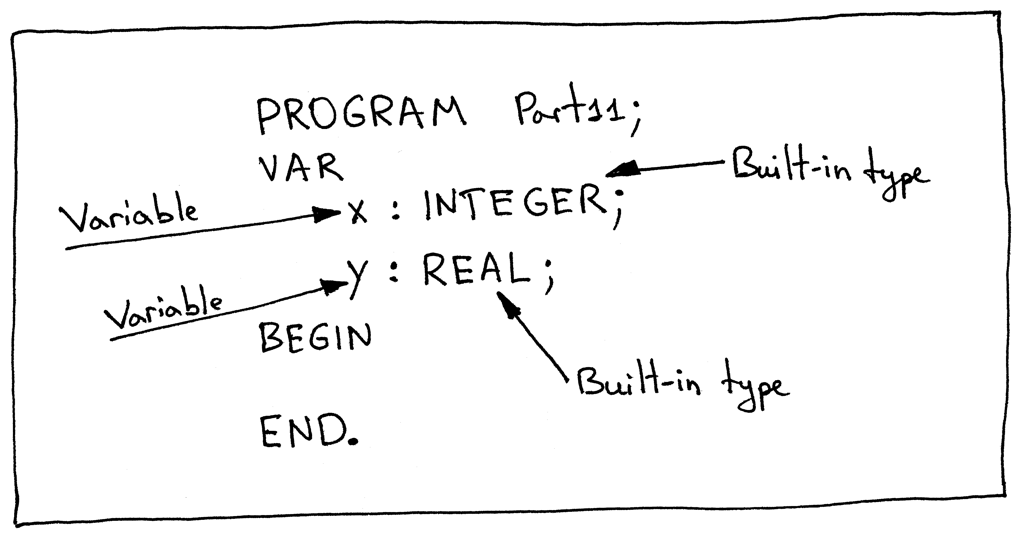``````class Symbol(object):
def __init__(self, name, type=None):
self.name = name
self.type = type
``````

``````class BuiltinTypeSymbol(Symbol):
def __init__(self, name):
super(BuiltinTypeSymbol, self).__init__(name)

def __str__(self):
return self.name

__repr__ = __str__
``````

``````\$ python
>>> from spi import BuiltinTypeSymbol
>>> int_type = BuiltinTypeSymbol('INTEGER')
>>> int_type
INTEGER
>>> real_type = BuiltinTypeSymbol('REAL')
>>> real_type
REAL
``````

``````class VarSymbol(Symbol):
def __init__(self, name, type):
super(VarSymbol, self).__init__(name, type)

def __str__(self):
return '<{name}:{type}>'.format(name=self.name, type=self.type)

__repr__ = __str__
``````

``````\$ python
>>> from spi import BuiltinTypeSymbol, VarSymbol
>>> int_type = BuiltinTypeSymbol('INTEGER')
>>> real_type = BuiltinTypeSymbol('REAL')
>>>
>>> var_x_symbol = VarSymbol('x', int_type)
>>> var_x_symbol
<x:INTEGER>
>>> var_y_symbol = VarSymbol('y', real_type)
>>> var_y_symbol
<y:REAL>
``````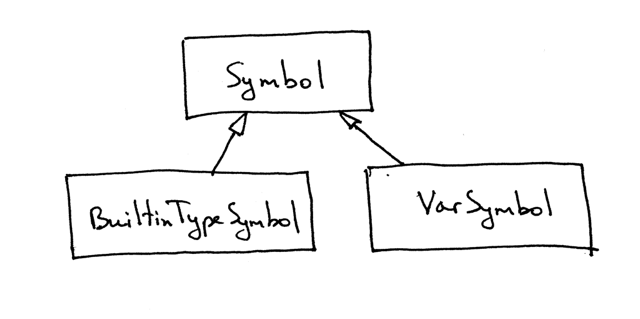• 为了确保当我们为变量赋值时，类型是正确的（类型检查）
• 确保变量在使用前被声明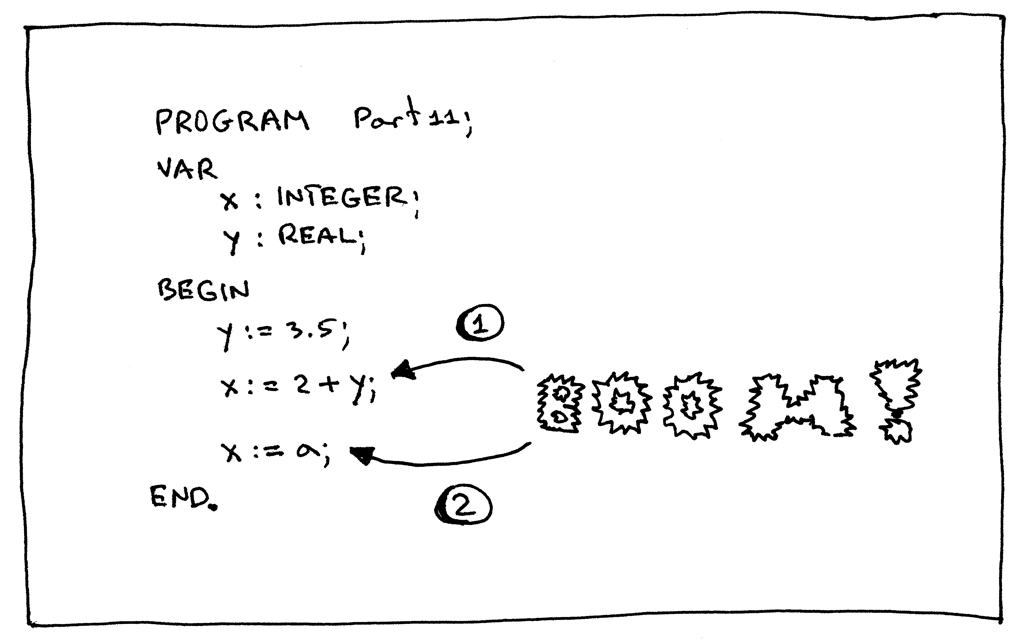1. 在表达式 “x := 2 + y;” 中，我们为被声明为整数的变量 “x” 赋予了一个小数值。 这不会编译，因为类型是不兼容的。
2. 在赋值语句 “x := a;” 中，我们引用了未声明的变量“a” —— 错误！

``````class SymbolTable(object):
def __init__(self):
self._symbols = OrderedDict()

def __str__(self):
s = 'Symbols: {symbols}'.format(
symbols=[value for value in self._symbols.values()]
)
return s

__repr__ = __str__

def define(self, symbol):
print('Define: %s' % symbol)
self._symbols[symbol.name] = symbol

def lookup(self, name):
print('Lookup: %s' % name)
symbol = self._symbols.get(name)
# 'symbol' is either an instance of the Symbol class or 'None'
return symbol
``````

``````PROGRAM Part11;
VAR
x : INTEGER;
y : REAL;

BEGIN

END.
``````

``````\$ python
>>> from spi import SymbolTable, BuiltinTypeSymbol, VarSymbol
>>> symtab = SymbolTable()
>>> int_type = BuiltinTypeSymbol('INTEGER')
>>> symtab.define(int_type)
Define: INTEGER
>>> symtab
Symbols: [INTEGER]
>>>
>>> var_x_symbol = VarSymbol('x', int_type)
>>> symtab.define(var_x_symbol)
Define: <x:INTEGER>
>>> symtab
Symbols: [INTEGER, <x:INTEGER>]
>>>
>>> real_type = BuiltinTypeSymbol('REAL')
>>> symtab.define(real_type)
Define: REAL
>>> symtab
Symbols: [INTEGER, <x:INTEGER>, REAL]
>>>
>>> var_y_symbol = VarSymbol('y', real_type)
>>> symtab.define(var_y_symbol)
Define: <y:REAL>
>>> symtab
Symbols: [INTEGER, <x:INTEGER>, REAL, <y:REAL>]
``````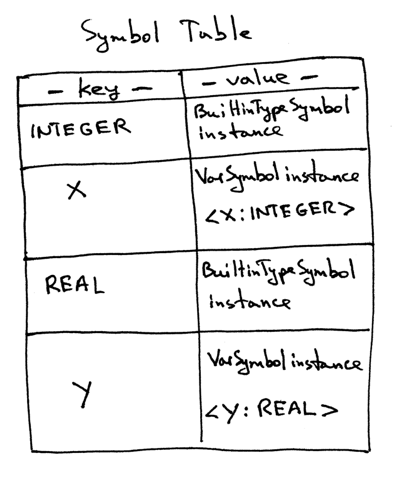``````class SymbolTable(object):
def __init__(self):
self._symbols = OrderedDict()
self._init_builtins()

def _init_builtins(self):
self.define(BuiltinTypeSymbol('INTEGER'))
self.define(BuiltinTypeSymbol('REAL'))

def __str__(self):
s = 'Symbols: {symbols}'.format(
symbols=[value for value in self._symbols.values()]
)
return s

__repr__ = __str__

def define(self, symbol):
print('Define: %s' % symbol)
self._symbols[symbol.name] = symbol

def lookup(self, name):
print('Lookup: %s' % name)
symbol = self._symbols.get(name)
# 'symbol' is either an instance of the Symbol class or 'None'
return symbol
``````

``````class SymbolTableBuilder(NodeVisitor):
def __init__(self):
self.symtab = SymbolTable()

def visit_Block(self, node):
for declaration in node.declarations:
self.visit(declaration)
self.visit(node.compound_statement)

def visit_Program(self, node):
self.visit(node.block)

def visit_BinOp(self, node):
self.visit(node.left)
self.visit(node.right)

def visit_Num(self, node):
pass

def visit_UnaryOp(self, node):
self.visit(node.expr)

def visit_Compound(self, node):
for child in node.children:
self.visit(child)

def visit_NoOp(self, node):
pass

def visit_VarDecl(self, node):
type_name = node.type_node.value
type_symbol = self.symtab.lookup(type_name)
var_name = node.var_node.value
var_symbol = VarSymbol(var_name, type_symbol)
self.symtab.define(var_symbol)
``````

``````def visit_VarDecl(self, node):
type_name = node.type_node.value
type_symbol = self.symtab.lookup(type_name)
var_name = node.var_node.value
var_symbol = VarSymbol(var_name, type_symbol)
self.symtab.define(var_symbol)
``````

``````\$ python
>>> from spi import Lexer, Parser, SymbolTableBuilder
>>> text = """
... PROGRAM Part11;
... VAR
...    x : INTEGER;
...    y : REAL;
...
... BEGIN
...
... END.
... """
>>> lexer = Lexer(text)
>>> parser = Parser(lexer)
>>> tree = parser.parse()
>>> symtab_builder = SymbolTableBuilder()
Define: INTEGER
Define: REAL
>>> symtab_builder.visit(tree)
Lookup: INTEGER
Define: <x:INTEGER>
Lookup: REAL
Define: <y:REAL>
>>> # Let’s examine the contents of our symbol table
…
>>> symtab_builder.symtab
Symbols: [INTEGER, REAL, <x:INTEGER>, <y:REAL>]
``````

``````def visit_Assign(self, node):
var_name = node.left.value
var_symbol = self.symtab.lookup(var_name)
if var_symbol is None:
raise NameError(repr(var_name))

self.visit(node.right)

def visit_Var(self, node):
var_name = node.value
var_symbol = self.symtab.lookup(var_name)

if var_symbol is None:
raise NameError(repr(var_name))
``````

``````PROGRAM NameError1;
VAR
a : INTEGER;

BEGIN
a := 2 + b;
END.
``````

``````\$ python
>>> from spi import Lexer, Parser, SymbolTableBuilder
>>> text = """
... PROGRAM NameError1;
... VAR
...    a : INTEGER;
...
... BEGIN
...    a := 2 + b;
... END.
... """
>>> lexer = Lexer(text)
>>> parser = Parser(lexer)
>>> tree = parser.parse()
>>> symtab_builder = SymbolTableBuilder()
Define: INTEGER
Define: REAL
>>> symtab_builder.visit(tree)
Lookup: INTEGER
Define: <a:INTEGER>
Lookup: a
Lookup: b
Traceback (most recent call last):
...
File "spi.py", line 674, in visit_Var
raise NameError(repr(var_name))
NameError: 'b'
``````

``````PROGRAM NameError2;
VAR
b : INTEGER;

BEGIN
b := 1;
a := b + 2;
END.
``````

``````>>> from spi import Lexer, Parser, SymbolTableBuilder
>>> text = """
... PROGRAM NameError2;
... VAR
...    b : INTEGER;
...
... BEGIN
...    b := 1;
...    a := b + 2;
... END.
... """
>>> lexer = Lexer(text)
>>> parser = Parser(lexer)
>>> tree = parser.parse()
>>> symtab_builder = SymbolTableBuilder()
Define: INTEGER
Define: REAL
>>> symtab_builder.visit(tree)
Lookup: INTEGER
Define: <b:INTEGER>
Lookup: b
Lookup: a
Traceback (most recent call last):
...
File "spi.py", line 665, in visit_Assign
raise NameError(repr(var_name))
NameError: 'a'
``````

``````PROGRAM Part11;
VAR
x : INTEGER;
BEGIN
x := 2;
END.
``````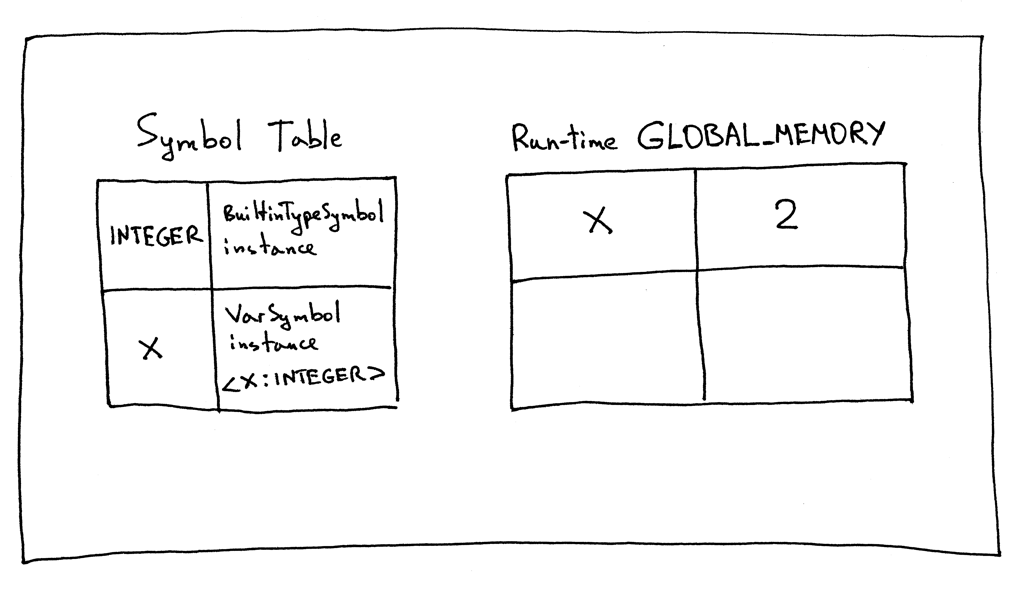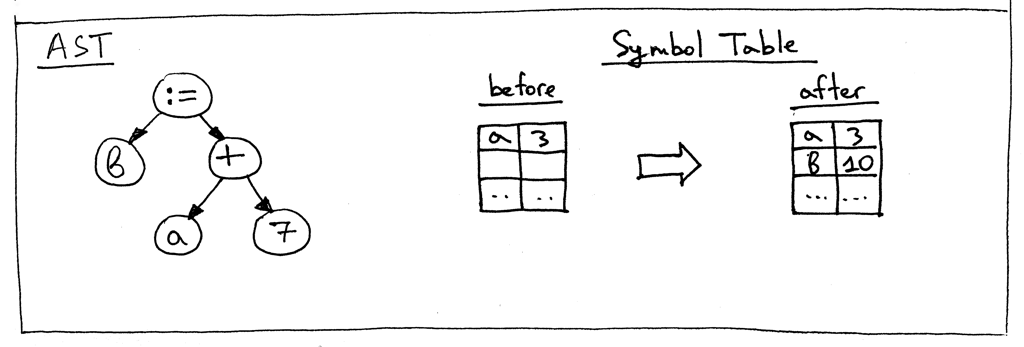``````PROGRAM Part11;
VAR
number : INTEGER;
a, b   : INTEGER;
y      : REAL;

BEGIN {Part11}
number := 2;
a := number ;
b := 10 * a + 10 * number DIV 4;
y := 20 / 7 + 3.14
END.  {Part11}
``````

``````\$ python spi.py part11.pas
Define: INTEGER
Define: REAL
Lookup: INTEGER
Define: <number:INTEGER>
Lookup: INTEGER
Define: <a:INTEGER>
Lookup: INTEGER
Define: <b:INTEGER>
Lookup: REAL
Define: <y:REAL>
Lookup: number
Lookup: a
Lookup: number
Lookup: b
Lookup: a
Lookup: number
Lookup: y

Symbol Table contents:
Symbols: [INTEGER, REAL, <number:INTEGER>, <a:INTEGER>, <b:INTEGER>, <y:REAL>]

Run-time GLOBAL_MEMORY contents:
a = 2
b = 25
number = 2
y = 5.99714285714
``````

• 什么是符号？
• 为什么我们需要跟踪符号？
• 什么是符号表？
• 定义符号和解析/查找符号有什么区别？
• 给定下面这个小的Pascal程序，符号表的内容是什么，全局内存呢？（GLOBAL_MEMORY字典是解释器的一部分）

``````PROGRAM Part11;
VAR
x, y : INTEGER;
BEGIN
x := 2;
y := 3 + x;
END.
``````P.S：我对符号和符号表管理主题的解释深受Terence Parr的《Language Implementation Patterns》一书影响。这是一本了不起的书。我认为它是我所见过的对符号表最清晰的解释，它也涵盖了类范围，这是我不打算在本系列中讨论的一个话题，因为我们不会讨论面向对象的Pascal。

P.P.S .:如果你等不及想开始深入了解编译器，我强烈推荐Jack Crenshaw的免费经典 “Let’s Build a Compiler.”